Guide To Simplifying Fractions And Improper Fractions

This article will show you how to simplify fractions and improper fractions.I am sure you are already familiar with the concept of fractions but let’s have a brief look at them anyway to refresh your memory!

Meanwhile, if you just want to use our fraction calculator please go right ahead.

Imagine your mother made a delicious pie that is now going to be divided between you and your siblings.If you have 3 other siblings, the pie will be divided into 4 equal parts and you will get to eat one part out of the four. This means, you ate 1/4th of the pie! Now, ¼ is a fraction and to put it simply, a fraction describes the number of parts of a certain size. (Remember: those parts have to be all equal in size!).If the pie is divided into 6 equal portions then one portion will be 1/6th of the pie. And two portions will be 2/6th of the pie. Now that we have refreshed the concept of fractions lets move a little further and see how some fractions can be simplified.

Moving back to the pie your mother made that was divided into four portions, imagine one of your siblings does not like it and has given you his portion, what fraction of the pie did you eat?

That’s right! 2/4th!

Here comes the tricky part. Two portions out of four is the same as one portion out two! Confusing? Imagine the pie is divided into two portions instead of four, and you get one of those two portions i.e ½ of the pie. This is the same portion if the pie was divided into four portions and you had two of them i.e. 2/4th of the pie.This means that:

Simplifying fractions means reducing the numerator (top number of fraction) and denominator (lower number of the fraction) to lowest possible numbers. This is important as it makes calculations involving fractions much easier as we will see later on in other articles.

Now that you can visualize what this whole ‘simplifying fractions’ concept means, lets move onto the mathematical techniques used to simplify the fractions.

For example, we have a fraction:

In this fraction, 5 is the numerator and 25 is the denominator. To simplify this fraction, we need to find the greatest number by which both numerator and denominator are divisible. In other words, we need to find the greatest common factor (GCF) of 5 and 25. If you don’t know how to do that, look at our article on ‘Finding GCF and LCM’ and we will also briefly explain it here:

Step 1: Write the prime factors of both numerator and denominator:

Step 2: Identify the numbers that appear in factors of both numbers: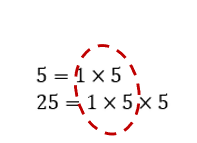Step 3: Multiply those factors identified in the step two to get GCF:

Step 4: Divide both the numerator and denominator by the GCF to get the simplified fraction:

So,

Simplifying Proper Fractions

Notice that in all the examples we have looked at so far, the numerator is smaller than the denominator. This type of fraction is called a ‘Proper Fraction’.

Let’s do some other examples:

Simplify:

Step1:

Step 2: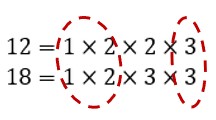Step 3:

Step 4:

So,

Simplifying Improper Fractions

Sometimes we come across improper fractions where the numerator is greater than the denominator. All the steps of simplifying proper fraction also apply to simplifying improper fractions, but in the end usually you are required to turn an improper fraction into a mixed number. Let’s see how that works:

Simplify:

Follow Step 1 to Step 4 as explained above:

Step1:

Step 2: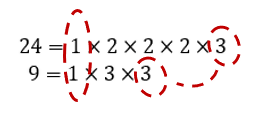Step 3:

Step 4:

The answer is still an improper fraction (Simplification of an improper fraction always gives an improper fraction) and in most of the cases the final answer of simplification of an improper fraction must be in mixed number form. We will explain how to do that in Step 5

Step 5:

Convert the fraction to a mixed number. In order to do that follow the following mini steps:

Mini step 5.1: Divide the numerator by denominator. The numerator is the divisor and the denominator is the dividend: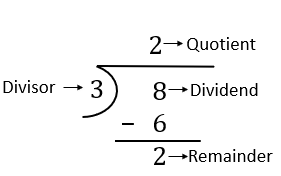Mini step 5.2: Write as mixed number in the following format:

This means that the fraction in our example can be written in mixed number form as:

Let’s do another example of simplifying an improper fraction:

Simplify:

Try to do it yourself before going through the solution!

Well? Did you get it right? Go through the solution below to check you result.

Step 1:

Step 2: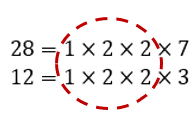Step 3:

Step 4:

Step 5: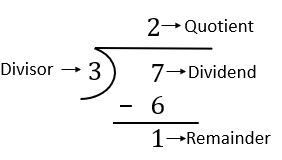Final answer in mixed number form is:

In this article we covered the concept of how to reduce fractions to their simplest form. Steps involved in simplifying both proper and improper fractions are the same except that final answer of improper fractions is usually desired to be in mixed number form.

Simplifying Mixed Number

We have covered everything there is to know about fraction simplification except one thing: What if you are given a mixed number and asked to simplify it? It is easy! lets see how to do it.

Simplify Remember that the fractional part of a mixed number is always a proper fraction. To simplify a mixed number, just simplify the fractional part of it and rewrite the mixed number.

For the example above simplify :

Step1:

Step 2: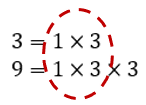Step 3:

Step 4: